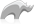##### [ percent ]

Type

Casting a [decimal] value as [percent] type formats the number in percent form.

This type is primarily used for display purposes.

Note: When cast as a [percent] type decimal values are first rounded to the nearest hundredth.

• Syntax
• Methods
• Traits
``percent( decimal )``
###### Has methods:
• `percent->oncreate(VALUE::decimal)`
• `percent->VALUE()`
• `percent->value=(VALUE)`
###### Has traits:
• `any`
• `formattingbase`
• `trait_asString`
• Beginner

### Display a decimal as a percent

Cast the [decimal] value as a [percent] type. This example shows the difference in display between various values cast both as decimal and as percent.

Code

``````var( myValues = array( .02, .135, 2.5, .004, .006 ) )

'Decimal\t\t%\n'
with v in \$myValues
do => {^
local( p = percent(#v) )
#v+'\t\t'+#p+'\n'
^}``````

Result

```Decimal		%
0.020000		2%
0.135000		14%
2.500000		250%
0.004000		0%
0.006000		1%```

### Display a decimal as a percent

Cast the [decimal] value as a [percent] type. This example shows the difference in display between various values cast both as decimal and as percent.

Code

``````var( myValues = array( .02, .135, 2.5, .004, .006 ) )

'Decimal\t\t%\n'
with v in \$myValues
do => {^
local( p = percent(#v) )
#v+'\t'+#p+'\n'
^}``````

Result

```Decimal		%
0.020000	2%
0.135000	14%
2.500000	250%
0.004000	0%
0.006000	1%```Please note that periodically LassoSoft will go through the notes and may incorporate information from them into the documentation. Any submission here gives LassoSoft a non-exclusive license and will be made available in various formats to the Lasso community.